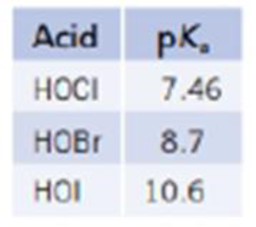Chapter 16, Problem 121SCQ

Chapter
Section
Textbook Problem

The halogens form three stable, weak acids, HOX.(a) Which is the strongest of these acids? (b) Explain why the acid strength changes as the halogen atom is changed.

(a)

Interpretation Introduction

Interpretation:

The strongest halogen acid has to be identified.

Concept Introduction:

The acidity of halogen acids is assigned according to the given pKa values. Lower the value of pKa for an acid, stronger is the acid. The acidic strength of the halogen acids increases as the electronegativity of the halogen atom increases.

Explanation

The value of pKa for given acids are listed in the table below

AcidpKaHOCl7.46HOBr8.7HOI10.6

The value of pKa is inversely proportional to the acidity

(b)

Interpretation Introduction

Interpretation:

The reason has to be explained for the change of the acidic order of halogen acids when changing halogen atoms.

Concept Introduction:

The acidity of halogen acids is assigned according to the given pKa values. Lower the value of pKa for an acid, stronger is the acid. The acidic strength of the halogen acids increases as the electronegativity of the halogen atom increases.

Still sussing out bartleby?

Check out a sample textbook solution.

See a sample solution

The Solution to Your Study Problems

Bartleby provides explanations to thousands of textbook problems written by our experts, many with advanced degrees!

Get Started

___ is the actual path length. (2.2)

An Introduction to Physical Science

Explain how Kapler’s lows contradict uniform circular motion.

Horizons: Exploring the Universe (MindTap Course List)

What is the function of bile salts in the digestion of fats?

Introduction to General, Organic and Biochemistry

How does binge-eating disorder differ from bulimia nervosa?

Nutrition Through the Life Cycle (MindTap Course List)